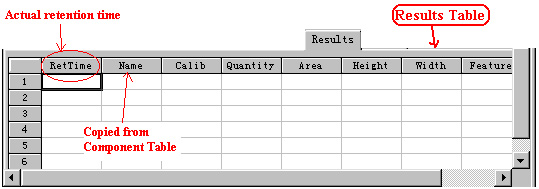Results of Analysis / Results Table
 ◇After activating the Calculating command, you can go to the Results Table to view the results of the calculation. When calculating the calibrators of a Standard sample, the calculated calibrators would be shown in the Calib (calibrator) column. When calculating components quantities of an unknown sample, the calculated quantities would be shown in the Quantity (quantity) column.